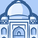App Development

Announcements
QLIKWORLD LIVE! MAY 16 - 19TH, EARLY BIRD DISCOUNTS! REGISTER TODAY
cancel
Showing results for
Did you mean:Contributor III

function in a set analysis expression

Hy everybody,

I want to use a syntax function in a set analysis expression :

Want to put my dimension upper() to have always maj caracter like this :

sum( {\$} < upper([DESCRIPTION]) not equal {"OK"} > isnull([DATE CHECK] )

1st pb -> function upper doesn't work like this

2nd one > how to write <> / != not/ not equal in a set analysis expression  ? Like this -= ?

Thanks a lot,

1 Solution

Accepted SolutionsMVP

Try like:

sum( {\$ < DESCRIPTION={"=upper([DESCRIPTION]) <> 'OK' "} >} isnull([DATE CHECK] ))

4 RepliesMVP

Try like:

sum( {\$ < DESCRIPTION={"=upper([DESCRIPTION]) <> 'OK' "} >} isnull([DATE CHECK] ))MVP

Double quotes around a value indicate a case-insensitive search.

Not equal is = - .

So:

sum( {\$ < DESCRIPTION = -{"OK"} >} isnull([DATE CHECK] ))Creator

@rwunderlichJust like Rob indicates.Contributor III
Author

Thanks a lot ,

and can I change now the value 'OK' by a flexible value ? for example with an another subject

count({<[PAYMENT DATE] = {"=year([PAYMENT DATE])= '2020' "}>} distinct [DOC NUMBER]) NOT 2020 but max(year([PAYMENT DATE]))

count({<[PAYMENT DATE] = {"=year([PAYMENT DATE])=  max(year([PAYMENT DATE])) "}>} distinct [ID]) ?Tags
Community Browser